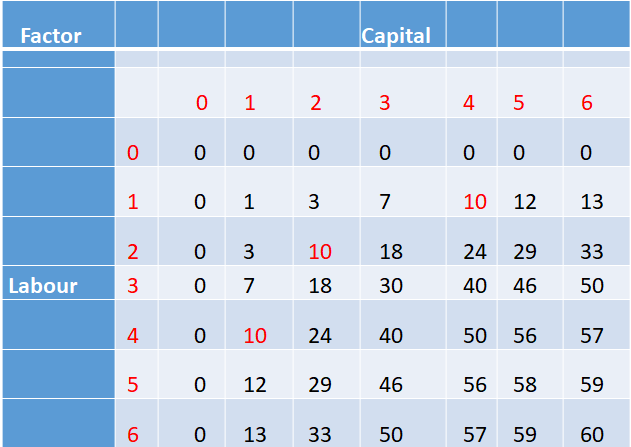Economics (NCERT) Notes → Class XII

3.7 Various Concepts of Costs

Cost Function•In order to produce output, the firm needs to employ inputs.
•A given level of output can be produced in many ways.
•A firm chooses the combination of inputs which is least expensive.
•Thus the cost function describes the least cost of producing each level of output given prices of factors of production and technology.

Cobb-Douglas Production Function
•Consider a production function x1 a x2 b, where a and b are constants.
•The firm produces amount of output using x1 amount of factor 1 and x2 amount of factor 2.
•This is called a Cobb-Douglas production function.

Production Function and Returns to Scale
•Let the production function be x1 a x2 b
•If we increase both the inputs (t > 1) times, we get the new output
q2 = (tx1) a (tx2b
q2 = t a+b (q)
•When a + b = 1, we have qtq. That is, the output increases times. So the production function exhibits Constant returns to scale (CRS) CRS.
•Similarly, when a + b 1, the production function exhibits Increasing returns to scale (IRS).
•When a + b 1 the production function exhibits Decreasing returns to scale (DRS).

Total variable cost (TVC).
•In the short run, some of the factors of production cannot be varied, and remain fixed.
•The cost that a firm incurs to employ these fixed inputs is called the total fixed cost (TFC).
•To produce any required level of output, the firm can adjust only variable inputs.
•Accordingly, the cost that a firm incurs to employ these variable inputs is called the total variable cost (TVC).

Total Cost
•It is obtained by adding the fixed and the variable costs.
•The total cost (TC) of a firm TC TVC TFC
•In order to increase the production of output, the firm must employ more of the variable inputs, which will also increase total cost.
•Therefore, as output increases, total variable cost and total cost increase.

Various Concepts of Costs
•The short run average cost (SAC) incurred by the firm is defined as the total cost per unit of output.
•SAC TC/q
•The average variable cost (AVC) is defined as the total variable cost per unit of output.
•AVC TVC/q
•Average fixed cost (AFC)
•AFC TFC/q
Thus SAC AVC AFC

Short run marginal cost (SMC)
•The short run marginal cost (SMC) is defined as the change in total cost per unit of change in output
SMC change in total cost/change in output
= ∆TC/ ∆q
where ∆ represents the change in the value of the variable

Various Concepts of Costs

Relationship between Average and Marginal Cost
•For any level of output, the sum of marginal costs up to that level gives us the total variable cost at that level.
•Average variable cost at some level of output is the average of all marginal costs up to that level.

 Related Articles
 • 3.2 Inequality in Poverty • 2.3 The Problem of Unemployment • 2.2 Quality of Population • 2.1 People as Resource • 1.2 Increasing Agricultural Productivity • 5.4 Case Studies of Consumer Rights • 5.3 Consumer Protection Act • 5.1 Consumer Rights • 4.4 Impact of Globalization in India • 4.2 Foreign Trade and Integration of Markets
 Recent Articles
 • “Poverty anywhere is a threat to prosperity everywhere”  • GDP along with GNH as the indices for measuring progress • Nobel Peace Prize, 2020 • Convention on the conservation of migratory species of wild animals India • Emerging Technologies Division set up • Decade of Healthy Ageing • The relevance of Gandhi in modern times  • Hyper Globalism: a threat to human prosperity? • Business Process Outsourcing in India: a booming phenomenon?  • Covid-19 and the BioMedical Waste crisis